+ +

# 13. Thick lens IOL power calculation

We have previously established a formula for calculating the power of IOL in a thin lens model, which did not take into account the design of the IOL (Coddington factor, central thickness), nor the characteristics specific to the cornea (impact of the posterior surface). This page is dedicated to developing an IOL power calculation formula in a thick lens eye model.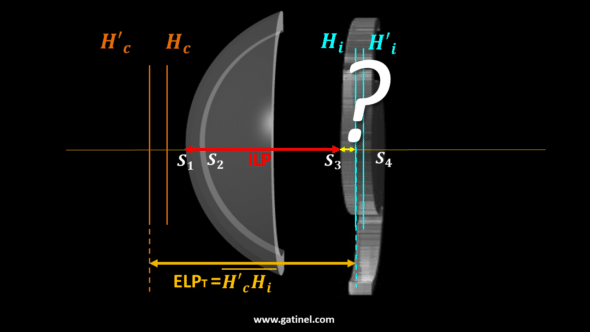## Preliminary considerations

Certain considerations are necessary before undertaking the calculation steps necessary to predict the power of an implant in a preoperative setting.

A schematic paraxial thick lens eye model is represented as an optical system combining the cornea and the IOL, with each ‘lens’ being defined by its anterior and posterior radius of curvature, its refractive index, and its thickness. In the context of a biometric power calculation for phakic eyes, we do not know by definition the power of the IOL that one must insert to reach the desired refractive target (this is what we are trying to predict! ). The position the IOL (its precise distance from the cornea) after surgery is also not known in advance.

In a thin lens eye model, the effective lens position (ELP) is usually defined as the distance from the anterior corneal surface to an infinitely thin IOL principal plane.

In a thick lens eye model,  one must distinguish the physical position of the IOL from its « optical » position.

In the paraxial approximation using thick lenses, a thick IOL optical position is referred to as the Effective Lens Position (ELPT, T for « thick »). It corresponds to the distance between the principal image plane of the cornea and the principal object plane of the IOLThis value depends not only on the physical position of the IOL in the eye but also on corneal thickness and IOL geometry. The latter varies between IOL models and powers.

We can define the Actual lens position (ALP)  as the physical distance measured from the anterior corneal surface to the anterior IOL surface.

We can also define the internal lens position (ILP) as the physical distance between the posterior corneal surface and the anterior IOL surface.  This value is independent of corneal thickness and IOL geometry,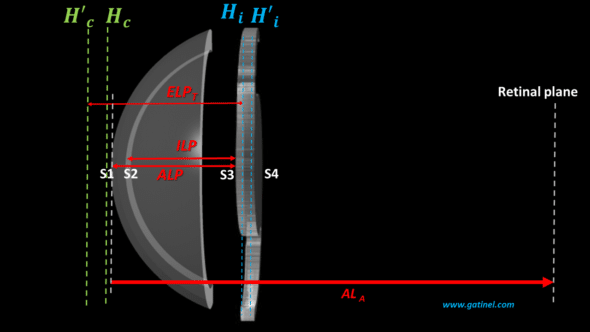## The formula for IOL power for targeting emmetropia (target SE =0)

Thanks to an emmetropic pseudophakic eye model equipped with thick refractive structures (cornea and IOL), we have established a general formula, which incorporates the essential elements for a biometric calculation:ALT and ELPT are a modified axial length and an effective position of the IOL within a thick lens model framework.

This expression also depends on the value of the corneal power (Dc), the power of the implant (Di), and those of the refractive indices considered (na, nv).

The above expression refers to an emmetropic eye. All we need is to solve it for the IOL power (Di).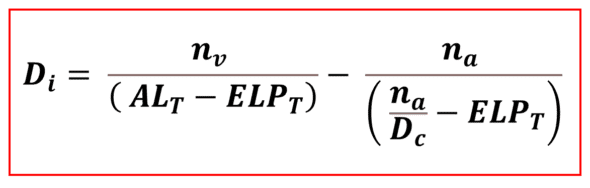This equation is the same as the one we obtained using the vergence formula applied to an eye model with thin lenses. This is expected, as the difference between the eye models with thin vs. thick lenses mainly relates to the difference between the appreciation of the axial length (ALA vs. ALT)  and effective lens position, i.e., the distance between the cornea and the IOL (ELP vs. ELPT).

We have established that the ALT value used in the formula differs slightly from the anatomical axial length (ALA). This is because we must subtract from the latter the distance between the implant’s principal planes, which we do not know in advance either! It is, however, possible to neglect this difference, which is quite minimal in practice, or else to carry out a first estimate of the power of the implant to obtain a plausible value of the ALT before redoing the calculation of the power of the implant.

We have obtained the formula giving  IOL power Di intended to make the eye emmetropic postoperatively. We will now study the case to aim for non-emmetropic postoperative refraction (i.e., an ametropia: usually mild myopia to achieve satisfactory uncorrected near visual acuity).

## The formula for IOL power for targeting ametropia (target SE ≠0)

The value of the IOL power  can be computed for a non-emmetropic target  (SE≠0) after replacing Dc by Dce the preceding equation, where Dce is the sum of Dc and the vergence in the corneal plane of a spectacle lens of power equal to SE, placed at distance d from the corneal vertex: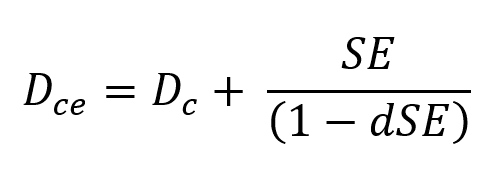We thus obtain the general formula for IOL power calculation aimed at inducing postoperative refraction equal to SE in the spectacle plane at distance d: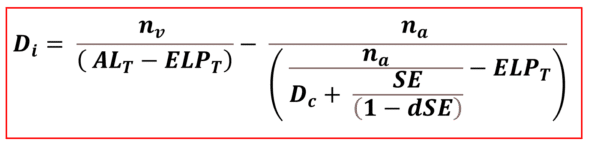To apply this formula and obtain a plausible result, a numeric value must be used for ELPT. However, we do not know this value in advance. In thick lenses, it depends on characteristics specific to the inserted IOL, including its power, precisely what we are trying to calculate.

## Thick IOL power calculation

The calculation of the implant’s power is carried out in two main steps, the first of which corresponds to the prediction of the value of the ELPT. This step is split into two sub-steps: the first aims to predict the ILP, and the second to calculate the distance separating the anterior surface from the IOL from its principal object plane. In the following figure, this distance corresponds to segment S3Hi.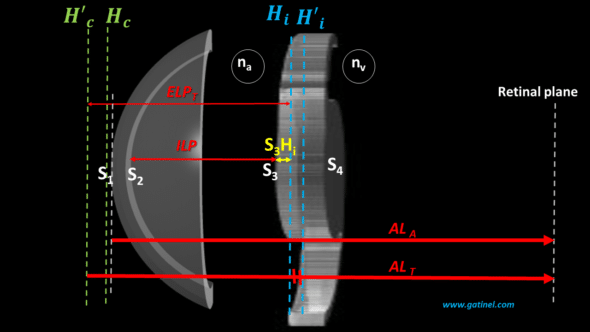The distance separating the main image plane of the cornea from the anterior surface of the cornea (H’cS1) is of the order of a few tens of microns and is negligible for the numerical calculation. However, its value can be calculated since it depends only on the biometric characteristics of the cornea.

### ILP Prediction

We had previously studied how it is possible, for an operated eye, to back-calculate the value of the ELPT, then deduce that of the ILP when we know the preoperative biometric parameters, the type, and the power of the IOLs used. If we repeat this procedure on a sufficient number of eyes, we can constitute a training set to design an AI algorithm or a regression formula.

With a training set made up of eyes for which the ILP is known and all the necessary biometric data, it was then possible to use an algorithmic method or even a regression method to predict ILP from preoperative biometric data.

Faced with a new eye, this algorithm predicts a plausible ILP value. However, it is still necessary to calculate S3Hi, the position of the principal object plane of an IOL, the power of which is still unknown at this stage (this is also what we are trying to predict).

### S3Hi prediction

The position of the principal object plane can be calculated using paraxial formulas, which use the geometric characteristics of the IOL  to be implemented. It is, however, necessary to know, among these variables, the power of the implant to be placed since this corresponds in return to particular anterior and posterior curvatures, as well as a certain central thickness.

A first step makes it possible to obtain an estimate of the IOL power intended to obtain the desired postoperative refraction (SE) by using as a first approximation the value of the ILP as the anatomical AND optical position of the IOL (as would occur if a thin lens pseudophakic eye model was used). Once this value has been obtained (1st iteration), and from the values of the Coddington factor (assumed to be known since we can select a known IOL model), IOL’s refractive index, and thickness, it is then possible to compute the value of S3Hi.

The predicted value of the ELPT is obtained by adding to the ILP the value of S3Hi (and to the segment H’cS1). It then becomes possible to make a second estimate of the power (2nd iteration), this time using the computed value for the predicted ELPT instead of that of ILP.

NB: This step also makes it possible to determine the value of the segment HiH’i, necessary for calculating the value ALT.

NB.2 : Implant power calculation formulas can be optimized by implementing a « constant », which is an « offset » allowing the predicted position of the implants to be shifted by the same value. The objective is to increase the accuracy of the formula by zeroing the average prediction error which can be appreciated after the first uses of the formula.

## Numerical computations

Using the spreadsheet below, we can calculate a theoretical value of the IOL power intended to induce target refraction (SE) postoperatively from preoperative biometric data. However, even if it provides realistic results, this online calculator should not be used because it has certain simplifications (see below) and has not been the subject of a clinical evaluation.

ILP corresponds to the anatomical position of the IOL: it was predicted as explained above using an algorithm trained with the preoperative biometric parameters as features.  In this example, this algorithm consisted of multiple linear regression, with the axial length, the anterior radius of curvature of the cornea, aqueous chamber depth, lens thickness, central corneal thickness (dc), and white-to-white diameter (WTW)  as independent variables. This value is used to obtain an approximate value of the power of the IOL to be inserted (1st iteration). From the power obtained by this first iteration, the value of the segments involved in calculating the ELPT and the ALT is calculated.

This value of the ELPT is then used to solve the equation again and obtain an IOL power value that considers the design characteristics of the latter in the context of a thick lens calculation. (2nd iteration).

As you will see using this spreadsheet, an isolated variation of the Coddington factor is likely to vary the power of the IOL required to reach the refractive target.

## A few remarks…

The calculation formula used for this spreadsheet assumes that the radius of the posterior surface of the cornea is known. This is often the case with modern biometers, but this value is not always measured, and therefore, it must be extrapolated. In thin-lens formulas, the total corneal power is inferred from the anterior radius of curvature, using the keratometric index. This method assumes that the relationship between the anterior and posterior radii radius is the same along the entire anterior corneal radius. Thus, when the posterior radius is not measured, one can use a previously determined anterior radius / posterior radius relationship.

Certain studies have shown that it is preferable to modify the value of the axial length to gain refractive precision. This reflects that modern biometric measurements, which rely on interferometric methods based on the optical path, do not always consider the slight differences between the refractive indices of the components of the eye. These variations result in a slight difference between the axial length extrapolated from a single refractive index value for the intraocular path and the axial length extrapolated from a segmentation of the eye as a function of the value of the local refractive index.

Once the predicted value of the ILP is obtained, it is also possible to use an approach consisting of calculating the theoretical refraction of the pseudophakic eye with a series of IOLs of increasing power and selecting the one whose power allows to achieve the refractive target. Finally, each of these implants is positioned so that its anterior face coincides with the predicted ILP position.

### 2 réponses à “13. Thick lens IOL power calculation”

1.Dr Damien Gatinel dit :

Thank you for your comments. The ELP in a thick lens model is defined as the distance between the cornea’s principal image plane and the IOL’s principal object plane. As the full details of the Barrett formula are not publicly available, I cannot make salient comments on the definition and concepts used for this formula and the definition of relevant segments. Regarding the IOL mater 700, I suggest you use the total corneal power for the IOL power calculation if you want to include the measured posterior surface of the cornea in your calculations. Else, you must convert the anterior and posterior astigmatism in vectors or orthogonal functions to perform the computation of resultant astigmatism. This is done automatically in some biometers using ray tracing or a similar approach.

2.Tai Yuan Che dit :

Hello , Dr Damien Gatinel ,

First , I want to thank you for your website ,which solve my question that bother me for years. I am an ophthalmologist working in Universal eye center , Taiwan.

We found that there is limitation in Barrett Universal II since the ELP is an estimated value.
Further more,the Barrett Universal II use Lens Factor to predict the ILP.
However he define the Lens Factor = distance between iris plane and secondary principle plane of IOL. Dose that mean Barrett define the ELP between S1 and Hi’?
According to your website, The ELP t is the distance between principle plane of image of cornea and principle plane of object of IOL(1st principle plane of IOL) .That confused me.

On the other hand,I have problem in entering the posterior cornea R. In our clinic ,we have IOL master 700 and the posterior R can be gained .However the anterior and posterior curvature of cornea are offered in different meridian.Since astigmatism are vectors,I can not just simply add them.How do you solve this problem ? Have you ask Zeiss to offer the cornea posterior radius in different meridians ? If I can get the value from all the meridians ,which meridian do you suggest to choose? (ex:the flat meridian) to calculate the IOL.

Sincerely yours Yuanche ,Tai

Warning: error_log(/home/clients/0e98f30bad73cbe357ff222deca9aba0/web/gatinel/wp-content/mc_data/53c4b42756475b28ee65f2b1a786a741-mc.log): failed to open stream: Disk quota exceeded in /home/clients/0e98f30bad73cbe357ff222deca9aba0/web/gatinel/wp-content/plugins/wpremote/protect/logger/fs.php on line 16